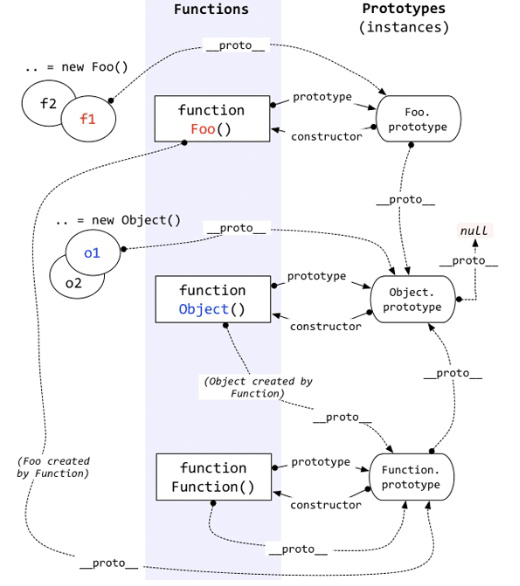``````function Foo(){}
var f1 = new Foo();
var f2 = new Foo();
``````

JavaScript基础进阶 : 基本类型与引用类型及其储存方式

``````function Foo(){}
f1 = new Foo();
f2 = new Foo();
console.log(f1.__proto__ === Foo.prototype);    //true
console.log(f2.__proto__ === Foo.prototype);    //true
````````````let a = {};    //  字面量形式
let a = new Object();    // 实例化Object构造函数
``````

prototype的用法：

``````function Foo(){}
Foo.prototype.name = 'dalao';
f1 = new Foo();
f2 = new Foo();
console.log(f1.name); // output: dalao
console.log(f2.name); // output: dalao
``````

``````function Foo(){}
f1 = new Foo();
f2 = new Foo();
console.log(Foo.prototype.constructor === Foo)    //true
console.log(f1.__proto__.constructor === Foo)    //true
console.log(f2.__proto__.constructor === Foo)    //true
``````

``````function Foo(){}
f1 = new Foo();
f2 = new Foo();
console.log(f1 === f2);    //false
console.log(f1.constructor === Foo);    //true
console.log(f2.constructor === Foo);    //true
console.log(f1.hasOwnProperty('constructor'))    //false
console.log(f2.hasOwnProperty('constructor'))    //false
``````

``````function Foo(){}
console.log(Foo.prototype.__proto__ === Object.prototype);    //true
``````

``````function Foo(){}
f1 = new Foo();
f2 = new Foo();
console.log(Object.prototype.constructor === Object);    //true
console.log(Foo.prototype.constructor === Foo);    //true
console.log(Foo.prototype.hasOwnProperty('constructor'))    //true
``````

``````console.log(Object.prototype.__proto__);    //null
``````

``````function Function(){}
Foo = new Function();
Object = new Function();
``````

``````function Foo(){}
f1 = new Foo();
f2 = new Foo();
console.log(Foo.__proto__ === Function.prototype);    //true
console.log(Object.__proto__ === Function.prototype);    //true
console.log(Array.__proto__ === Function.prototype);    //true
console.log(Foo.hasOwnProperty('constructor'));    //false
console.log(Foo.__proto__.hasOwnProperty('constructor'))    //true
``````

``````function Foo(){}
f1 = new Foo();
f2 = new Foo();
console.log(Foo.__proto__.constructor === Function);    //true
console.log(Foo.constructor === Function);    //true
console.log(Object.constructor === Function);    //true
console.log(Array.constructor === Function);    //true
``````

``````function Function(){}    //举例
Function = new Function();    //举例
console.log(Function.__proto__ === Function.prototype);    //true
console.log(Function.__proto__.constructor === Function);    //true
console.log(Function.constructor === Function);    //true
``````

constructor会覆盖掉继承的Object.prototype的属性，指向构造函数Function：

``````console.log(Function.prototype.__proto__ === Object.prototype);    //true
console.log(Function.prototype.constructor === Function)    //true
``````

Object.prototype作为实例对象时，它的__proto__属性指向null，也是一切皆对象的论点